# yitu

21. Mar 2023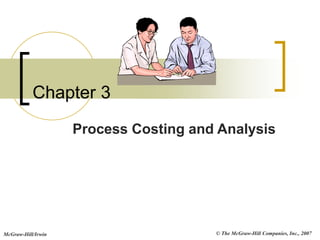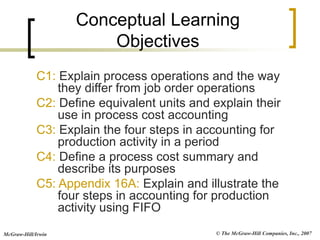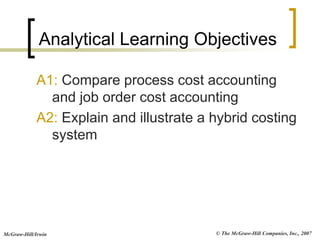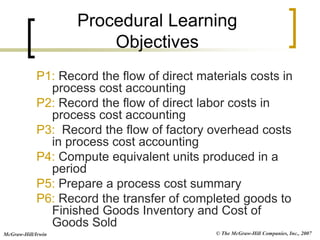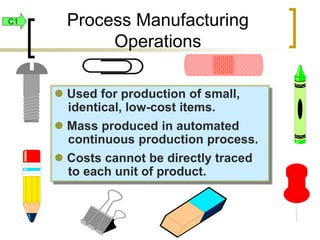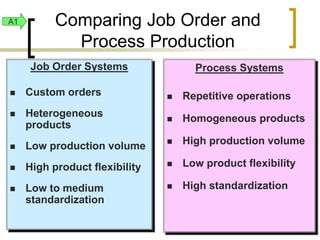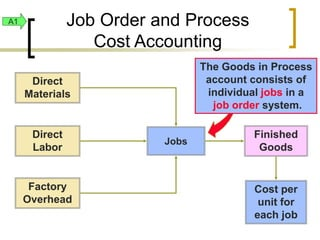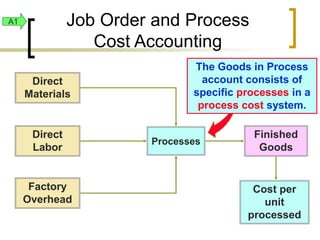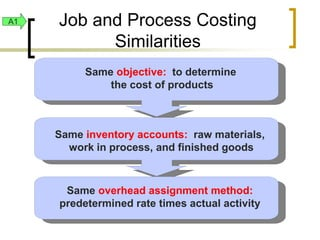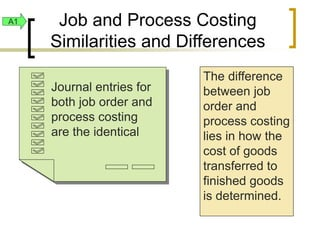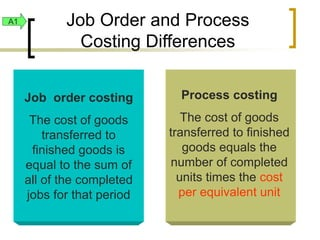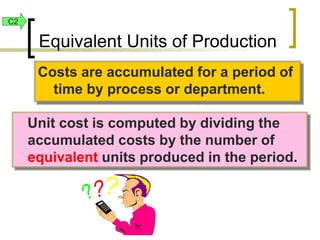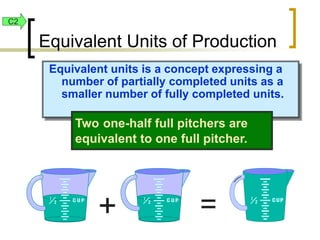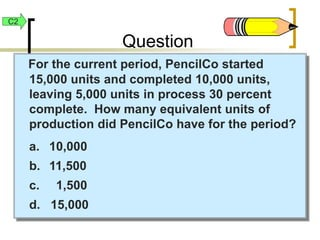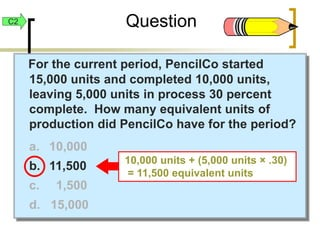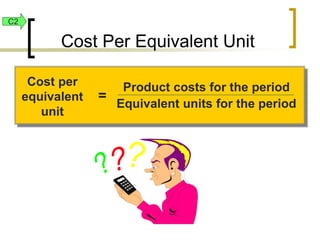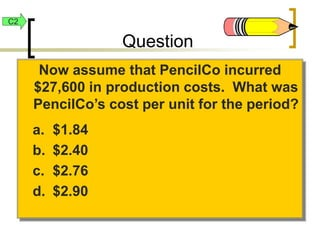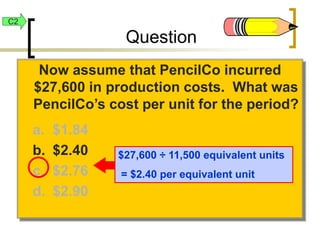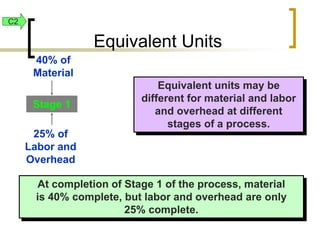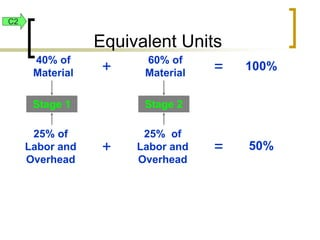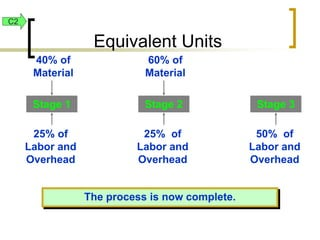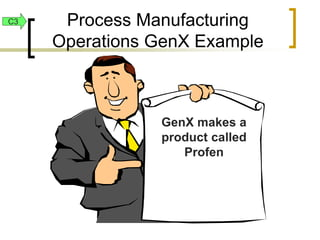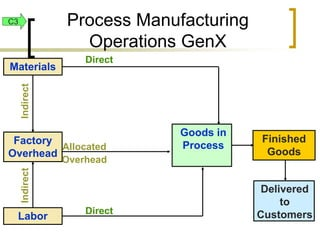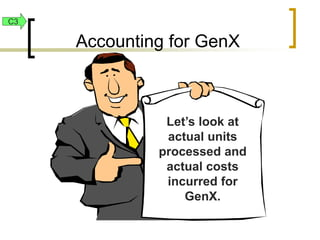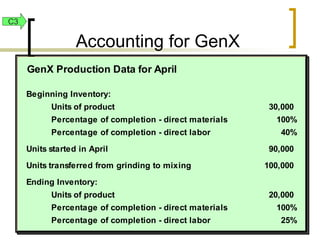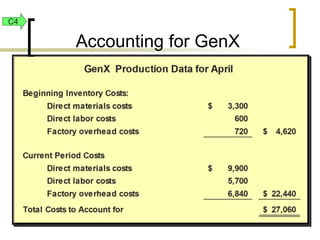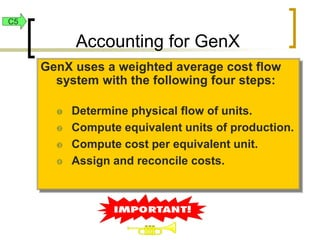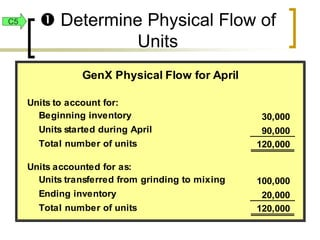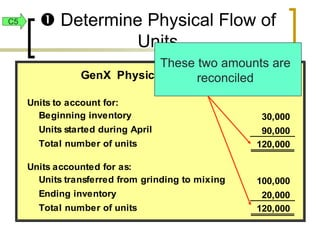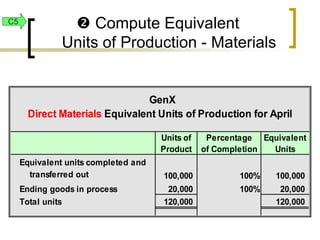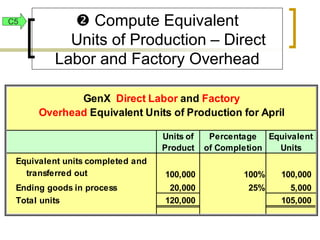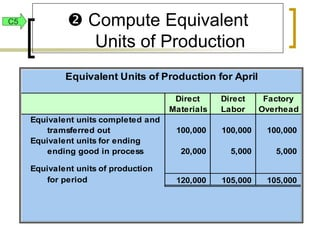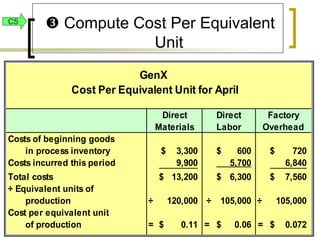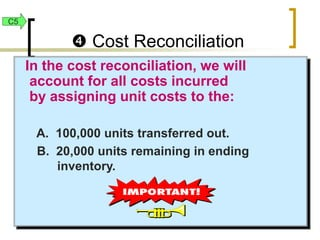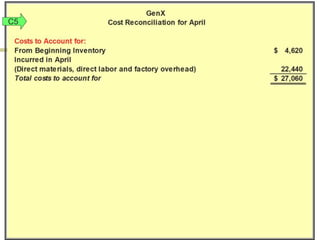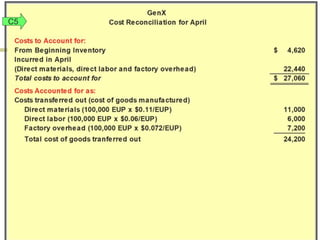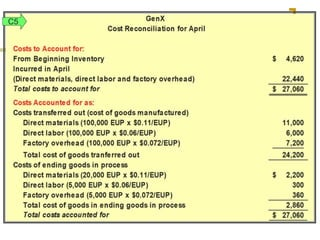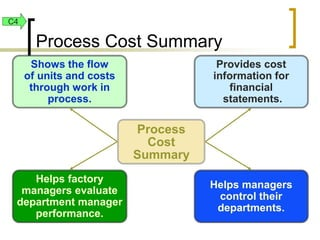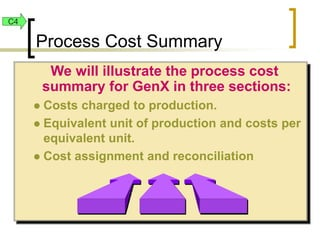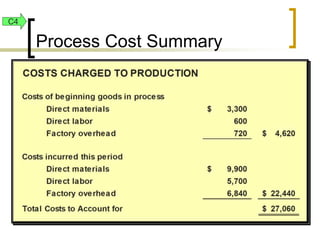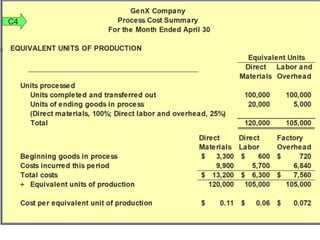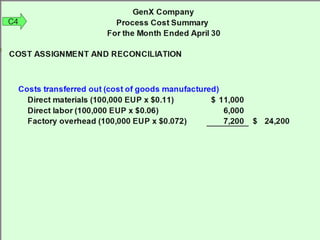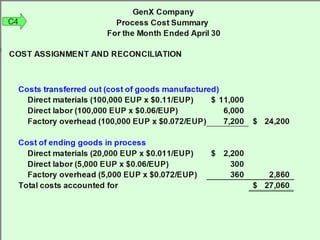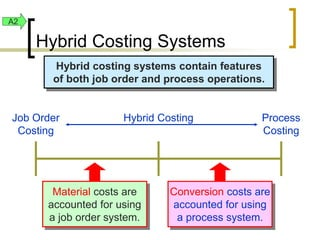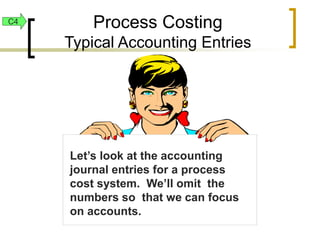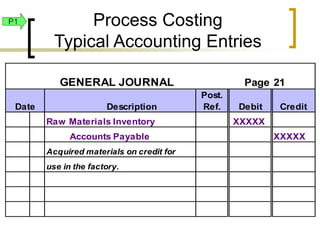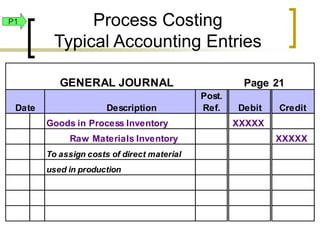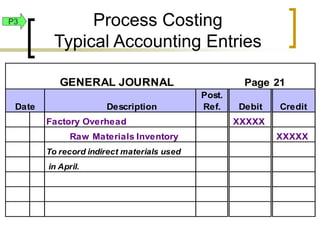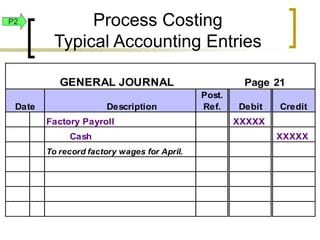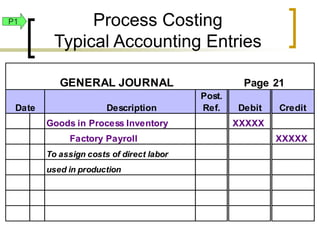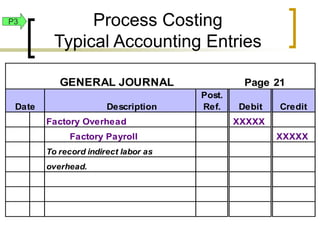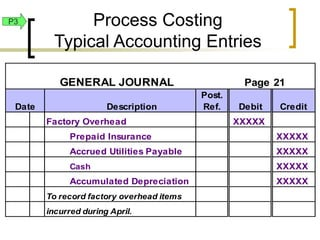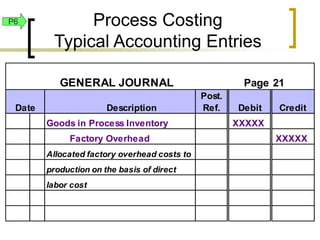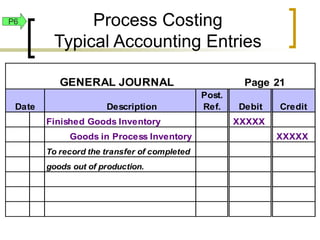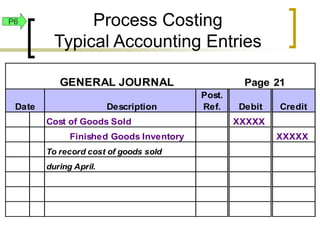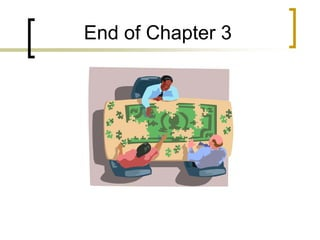1 von 56

### yitu

• 1. © The McGraw-Hill Companies, Inc., 2007 McGraw-Hill/Irwin Chapter 3 Process Costing and Analysis
• 2. © The McGraw-Hill Companies, Inc., 2007 McGraw-Hill/Irwin Conceptual Learning Objectives C1: Explain process operations and the way they differ from job order operations C2: Define equivalent units and explain their use in process cost accounting C3: Explain the four steps in accounting for production activity in a period C4: Define a process cost summary and describe its purposes C5: Appendix 16A: Explain and illustrate the four steps in accounting for production activity using FIFO
• 3. © The McGraw-Hill Companies, Inc., 2007 McGraw-Hill/Irwin A1: Compare process cost accounting and job order cost accounting A2: Explain and illustrate a hybrid costing system Analytical Learning Objectives
• 4. © The McGraw-Hill Companies, Inc., 2007 McGraw-Hill/Irwin P1: Record the flow of direct materials costs in process cost accounting P2: Record the flow of direct labor costs in process cost accounting P3: Record the flow of factory overhead costs in process cost accounting P4: Compute equivalent units produced in a period P5: Prepare a process cost summary P6: Record the transfer of completed goods to Finished Goods Inventory and Cost of Goods Sold Procedural Learning Objectives
• 5.  Used for production of small, identical, low-cost items.  Mass produced in automated continuous production process.  Costs cannot be directly traced to each unit of product. Process Manufacturing Operations C1
• 6. Job Order Systems  Custom orders  Heterogeneous products  Low production volume  High product flexibility  Low to medium standardization Process Systems  Repetitive operations  Homogeneous products  High production volume  Low product flexibility  High standardization Comparing Job Order and Process Production A1
• 7. Direct Materials Finished Goods Cost per unit for each job Direct Labor Factory Overhead Jobs The Goods in Process account consists of individual jobs in a job order system. Job Order and Process Cost Accounting A1
• 8. Direct Materials Finished Goods Direct Labor Factory Overhead Processes The Goods in Process account consists of specific processes in a process cost system. Cost per unit processed A1 Job Order and Process Cost Accounting
• 9. Same objective: to determine the cost of products Same inventory accounts: raw materials, work in process, and finished goods Same overhead assignment method: predetermined rate times actual activity Job and Process Costing Similarities A1
• 10. Job and Process Costing Similarities and Differences A1 Journal entries for both job order and process costing are the identical The difference between job order and process costing lies in how the cost of goods transferred to finished goods is determined.
• 11. A1 Job Order and Process Costing Differences Job order costing The cost of goods transferred to finished goods is equal to the sum of all of the completed jobs for that period Process costing The cost of goods transferred to finished goods equals the number of completed units times the cost per equivalent unit
• 12. Costs are accumulated for a period of time by process or department. Unit cost is computed by dividing the accumulated costs by the number of equivalent units produced in the period. Equivalent Units of Production C2
• 13. Equivalent units is a concept expressing a number of partially completed units as a smaller number of fully completed units. Two one-half full pitchers are equivalent to one full pitcher. + = Equivalent Units of Production C2
• 14. For the current period, PencilCo started 15,000 units and completed 10,000 units, leaving 5,000 units in process 30 percent complete. How many equivalent units of production did PencilCo have for the period? a. 10,000 b. 11,500 c. 1,500 d. 15,000 Question C2
• 15. For the current period, PencilCo started 15,000 units and completed 10,000 units, leaving 5,000 units in process 30 percent complete. How many equivalent units of production did PencilCo have for the period? a. 10,000 b. 11,500 c. 1,500 d. 15,000 10,000 units + (5,000 units × .30) = 11,500 equivalent units Question C2
• 16. Cost per equivalent unit = Product costs for the period Equivalent units for the period Cost Per Equivalent Unit C2
• 17. Now assume that PencilCo incurred \$27,600 in production costs. What was PencilCo’s cost per unit for the period? a. \$1.84 b. \$2.40 c. \$2.76 d. \$2.90 C2 Question
• 18. Now assume that PencilCo incurred \$27,600 in production costs. What was PencilCo’s cost per unit for the period? a. \$1.84 b. \$2.40 c. \$2.76 d. \$2.90 \$27,600 ÷ 11,500 equivalent units = \$2.40 per equivalent unit Question C2
• 19. Equivalent units may be different for material and labor and overhead at different stages of a process. At completion of Stage 1 of the process, material is 40% complete, but labor and overhead are only 25% complete. Stage 1 40% of Material 25% of Labor and Overhead Equivalent Units C2
• 20. Stage 2 25% of Labor and Overhead 60% of Material Stage 1 40% of Material 25% of Labor and Overhead + + = = 100% 50% Equivalent Units C2
• 21. Stage 3 50% of Labor and Overhead The process is now complete. Stage 2 25% of Labor and Overhead 60% of Material Stage 1 40% of Material 25% of Labor and Overhead Equivalent Units C2
• 22. GenX makes a product called Profen Process Manufacturing Operations GenX Example C3
• 23. Labor Materials Indirect Indirect Factory Overhead Direct Direct Delivered to Customers Goods in Process Finished Goods Allocated Overhead Process Manufacturing Operations GenX C3
• 24. Let’s look at actual units processed and actual costs incurred for GenX. Accounting for GenX C3
• 25. GenX Production Data for April Beginning Inventory: Units of product 30,000 Percentage of completion - direct materials 100% Percentage of completion - direct labor 40% Units started in April 90,000 Units transferred from grinding to mixing 100,000 Ending Inventory: Units of product 20,000 Percentage of completion - direct materials 100% Percentage of completion - direct labor 25% Accounting for GenX C3
• 26. C4 Accounting for GenX
• 27. GenX uses a weighted average cost flow system with the following four steps:  Determine physical flow of units.  Compute equivalent units of production.  Compute cost per equivalent unit.  Assign and reconcile costs. Accounting for GenX C5
• 28. GenX Physical Flow for April Units to account for: Beginning inventory 30,000 Units started during April 90,000 Total number of units 120,000 Units accounted for as: Units transferred from grinding to mixing 100,000 Ending inventory 20,000 Total number of units 120,000  Determine Physical Flow of Units C5
• 29. GenX Physical Flow for April Units to account for: Beginning inventory 30,000 Units started during April 90,000 Total number of units 120,000 Units accounted for as: Units transferred from grinding to mixing 100,000 Ending inventory 20,000 Total number of units 120,000  Determine Physical Flow of Units C5 These two amounts are reconciled
• 30. GenX Direct Materials Equivalent Units of Production for April Units of Percentage Equivalent Product of Completion Units Equivalent units completed and transferred out 100,000 100% 100,000 Ending goods in process 20,000 100% 20,000 Total units 120,000 120,000  Compute Equivalent Units of Production - Materials C5
• 31. GenX Direct Labor and Factory Overhead Equivalent Units of Production for April Units of Percentage Equivalent Product of Completion Units Equivalent units completed and transferred out 100,000 100% 100,000 Ending goods in process 20,000 25% 5,000 Total units 120,000 105,000  Compute Equivalent Units of Production – Direct Labor and Factory Overhead C5
• 32. Equivalent Units of Production for April Direct Direct Factory Materials Labor Overhead Equivalent units completed and tramsferred out 100,000 100,000 100,000 Equivalent units for ending ending good in process 20,000 5,000 5,000 Equivalent units of production for period 120,000 105,000 105,000  Compute Equivalent Units of Production C5
• 33. GenX Cost Per Equivalent Unit for April Direct Direct Factory Materials Labor Overhead Costs of beginning goods in process inventory 3,300 \$ 600 \$ 720 \$ Costs incurred this period 9,900 5,700 6,840 Total costs 13,200 \$ 6,300 \$ 7,560 \$ ÷ Equivalent units of production ÷ 120,000 ÷ 105,000 ÷ 105,000 Cost per equivalent unit of production = 0.11 \$ = 0.06 \$ = 0.072 \$  Compute Cost Per Equivalent Unit C5
• 34. In the cost reconciliation, we will account for all costs incurred by assigning unit costs to the: A. 100,000 units transferred out. B. 20,000 units remaining in ending inventory.  Cost Reconciliation C5
• 35. C5
• 36. C5
• 37. C5
• 38. Process Cost Summary Process Cost Summary Helps managers control their departments. Provides cost information for financial statements. Shows the flow of units and costs through work in process. Helps factory managers evaluate department manager performance. C4
• 39. We will illustrate the process cost summary for GenX in three sections:  Costs charged to production.  Equivalent unit of production and costs per equivalent unit.  Cost assignment and reconciliation Process Cost Summary C4
• 40. Process Cost Summary C4
• 41. C4
• 42. C4
• 43. C4
• 44. Hybrid costing systems contain features of both job order and process operations. Job Order Costing Process Costing Hybrid Costing Material costs are accounted for using a job order system. Conversion costs are accounted for using a process system. Hybrid Costing Systems A2
• 45. Let’s look at the accounting journal entries for a process cost system. We’ll omit the numbers so that we can focus on accounts. Process Costing Typical Accounting Entries C4
• 46. GENERAL JOURNAL Page 21 Date Description Post. Ref. Debit Credit Raw Materials Inventory XXXXX Accounts Payable XXXXX Acquired materials on credit for use in the factory. P1 Process Costing Typical Accounting Entries
• 47. GENERAL JOURNAL Page 21 Date Description Post. Ref. Debit Credit Goods in Process Inventory XXXXX Raw Materials Inventory XXXXX To assign costs of direct material used in production P1 Process Costing Typical Accounting Entries
• 48. GENERAL JOURNAL Page 21 Date Description Post. Ref. Debit Credit Factory Overhead XXXXX Raw Materials Inventory XXXXX To record indirect materials used in April. P3 Process Costing Typical Accounting Entries
• 49. GENERAL JOURNAL Page 21 Date Description Post. Ref. Debit Credit Factory Payroll XXXXX Cash XXXXX To record factory wages for April. P2 Process Costing Typical Accounting Entries
• 50. GENERAL JOURNAL Page 21 Date Description Post. Ref. Debit Credit Goods in Process Inventory XXXXX Factory Payroll XXXXX To assign costs of direct labor used in production P1 Process Costing Typical Accounting Entries
• 51. GENERAL JOURNAL Page 21 Date Description Post. Ref. Debit Credit Factory Overhead XXXXX Factory Payroll XXXXX To record indirect labor as overhead. P3 Process Costing Typical Accounting Entries
• 52. GENERAL JOURNAL Page 21 Date Description Post. Ref. Debit Credit Factory Overhead XXXXX Prepaid Insurance XXXXX Accrued Utilities Payable XXXXX Cash XXXXX Accumulated Depreciation XXXXX To record factory overhead items incurred during April. P3 Process Costing Typical Accounting Entries
• 53. GENERAL JOURNAL Page 21 Date Description Post. Ref. Debit Credit Goods in Process Inventory XXXXX Factory Overhead XXXXX Allocated factory overhead costs to production on the basis of direct labor cost P6 Process Costing Typical Accounting Entries
• 54. GENERAL JOURNAL Page 21 Date Description Post. Ref. Debit Credit Finished Goods Inventory XXXXX Goods in Process Inventory XXXXX To record the transfer of completed goods out of production. P6 Process Costing Typical Accounting Entries
• 55. GENERAL JOURNAL Page 21 Date Description Post. Ref. Debit Credit Cost of Goods Sold XXXXX Finished Goods Inventory XXXXX To record cost of goods sold during April. P6 Process Costing Typical Accounting Entries
• 56. End of Chapter 3

### Hinweis der Redaktion

1. This chapter focuses on how to measure and account for costs in process operations. We explain process production, describe how to assign costs to processes, and compute cost per equivalent unit for a process.
2. Process costing is used to determine the unit costs of small, low-cost items. We could not justify the use of job order costing for such small items.
3. On the left you see characteristics of a job order system that you learned in the previous chapter. Compare the characteristics of a process costing system on the right with those of a job order system. In a job order system, the focus is on the job, while in a process system, the focus is on the process.
4. Recall that in a job order system we trace direct material costs and direct labor costs to jobs. Factory overhead is an indirect cost that we allocate to jobs using a predetermined overhead rate.
5. In a process cost system, we trace direct material costs and direct labor costs to the process. Factory overhead is an indirect cost that we allocate to the process using a predetermined overhead rate. Once the costs are properly accumulated in the process, we will divide these costs by the units processed to get the cost per unit for the period.
6. There are a great number of similarities between job order and process costing, so we will use many of the same concepts we learned in Chapter 15 as we study process costing.
7. All of the journal entries – for the acquisition and use of raw materials, the acquisition and use of labor, the incurring and allocation of fixed overhead, and the transfer of completed goods from goods in process to finished goods – are identical. The difference lies in how to determine the cost of completed goods.
8. Direct material, direct labor, and factory overhead costs are accumulated for a process for a production period. Once the costs are properly accumulated in the process, we will divide these costs by the equivalent units processed to get the cost per unit for the period. The concept of equivalent units can cause some difficulty, so we will use several examples to illustrate its use in process cost systems.
9. Equivalent units is a term that refers to the number of units that would have been completed if all of the effort during a period had been applied only to the units started in that period. For example, four thousand units that are sixty percent complete would total two thousand four hundred equivalent units (sixty percent of four thousand is two thousand four hundred). Take a few minutes to answer the following two questions. They deal with calculating and using equivalent units. Check your progress on these concepts before moving on.
10. Here is the first of your two questions. .
11. The number of equivalent units is equal to the number of units completed plus the number of units remaining in ending goods in process times the completion percentage for those incomplete units.
12. Total production costs for a production period are divided by the equivalent units produced for the period to get the cost per unit.
13. Here is your second question.
14. Total production costs are divided by the equivalent units produced for the period to get the cost per unit.
15. If materials, labor and overhead are added at different rates in the process, the completion percentages will be different. Multiplying different percentages times the unfinished units will result in different equivalent units for materials, labor and overhead.
16. In this example, we see that material is added before labor and overhead, resulting in a higher completion percentage. This is not unusual as material must be present first before the work can begin. Think about baking cookies. All of the ingredients (materials) are present before the processing begins in the oven.
17. Once all of the labor and overhead are added to the materials in the process, the units are complete.
18. Let’s look at an example illustrating the flow of units and costs in a process system.
19. Direct materials and direct labor are traced to the processes. Factory overhead items are indirect and must be allocated to the process using a predetermined overhead rate.
20. Now let’s add numbers of units and dollars of costs to the example. We will examine the two processing departments in order of the production and cost flows, Grinding.first, and then Mixing.
21. Here we see the units processed and the completion percentages. Take a minute to become familiar with these numbers before moving on to the cost information. Process costing applications include lots of numbers and computations, so you may find yourself referring back to this given information as we work through the example.
22. The beginning inventory costs were incurred in March for the partially completed units left in ending inventory in Grinding. March’s ending inventory in Grinding becomes April’s beginning inventory. The current period’s costs were incurred in Grinding to complete the beginning inventory and to start additional units.
23. As with all situations involving inventory costs, we must choose a cost flow method. We will use the weighted average method for GenX,. Process cost applications can be overwhelming if we do not use a well-planned approach. The four-step procedure will enable us to solve a process cost application in manageable parts.
24. The beginning inventory of units plus the units started in April equal the total units to account for in the Grinding process for April. The total number of units is either completed and transferred to Mixing or is left partially completed in Grinding.
25. To convert units to equivalent units, we multiply by the appropriate percentage. Since the materials are added at the start of the period, the physical units ending goods in process have 100% of the materials attached to them.
26. Now that we have completed the equivalent units computations for material, we can move on to labor and overhead. We can compute equivalent units for labor and overhead at one time since both labor and overhead have the same completion percentages.
27. The equivalent units computation for material, labor, and overhead from the preceding computations are summarized in this table. Notice that the equivalent units for material differs from the equivalent units for labor and overhead because the completion percentages differed.
28. Costs for April are divided by the equivalent units for April to get the cost per equivalent unit.
29. Now that we have completed the computations for equivalent units and for costs per equivalent unit, we can use those results to compute the cost of the one hundred thousand units completed and transferred out and the cost of the partially completed units remaining in the process at the end of April.
30. We add the beginning inventory costs to the costs incurred for April to get the total costs to be accounted for in Grinding.
31. The cost of the units completed equal the cost of the 100,000 equivalent units of production for the direct materials, direct labor and factory overhead.
32. The ending inventory cost in Grinding is the sum of direct material, direct labor and factory overhead costs. The number of equivalent units for each product cost is multiplied by the respective unit cost. For example, the direct labor cost in ending inventory, three hundred dollars, is computed by multiplying five thousand equivalent units times 60 cents per unit. The total costs accounted for, twenty seven thousand sixty five dollars, is equal to the total costs to account for, twenty seven thousand sixty dollars.
33. The computations of equivalent units, unit costs, and cost assignments to units completed and transferred, and to units remaining in ending inventory are summarized in a report called the process cost summary. In addition to providing inventory costs for financial statements, this report helps managers evaluate performance and control department operations,.
34. The process cost summary has three parts: (1) costs charged to production; (2) computation of equivalent units and cost per equivalent unit; and (3) the cost assignment to unfinished units remaining in process and to units completed and transferred.
35. Costs charged to production for April include the costs in beginning inventory and the costs for direct material, direct labor, and factory overhead that are incurred in April.
36. The first portion of the Process Cost Summary summarizes the flow of units and the computation of equivalent units for each product cost. In the lower portion, you see the computation of cost per equivalent unit for each product cost for April.
37. Here we see the computation of the cost of the 100,000 units completed and transferred out.
38. The ending inventory cost is the sum of direct material, direct labor and factory overhead costs for the unfinished units. The number of equivalent units for each product cost is multiplied by the respective unit cost. For example, the factory overhead cost in ending inventory, three hundred sixty dollars, is computed by multiplying five thousand equivalent units times point zero seven two per unit. The total costs accounted for are twenty seven thousand sixty dollars, which is equal to the twenty seven thousand sixty dollars to account for.
39. Hybrid costing includes features of both job order and process costing. Material costs are traced directly as in job order costing, but conversion costs (labor and overhead) are accounted for in the same manner as used in process costing.
40. The following journal entries illustrate the recording process for a process cost system.
41. To record material purchased on account, we increase the raw materials inventory account with a debit entry and we increase accounts payable with a credit entry.
42. When direct material is used, we increase goods in process with a debit entry and decrease the raw materials inventory account with a credit entry.
43. When indirect materials are used, we charge the factory overhead account using a debit entry and decrease the raw materials inventory account with a credit entry.
44. When salaries and wages are paid, we record the amount in the factory payroll account with a debit entry and we reduce cash with a credit entry.
45. The portion of factory payroll that is classified as direct labor increases the goods in process inventory accounts which is accomplished with a debit entry.
46. The portion of factory payroll that is classified as indirect direct labor increases the factory overhead account, a debit entry.
47. Other actual costs for factory overhead items are recorded with a debit entry to the factory overhead account. Prepaid insurance is an asset account that is reduced with a credit as the insurance coverage is used. Accrued liabilities payable is a liability account that is increased with a credit. Cash is an asset account that is reduced with a credit indicating that cash has been paid. Accumulated depreciation is a contra asset account that is increased with a credit to reflect the adjusting entry for depreciation on factory buildings and equipment..
48. Factory overhead is applied to the process using a predetermined overhead rate, resulting in an increase in the goods in process inventory account.
49. Completed units are transferred from production to the finished goods inventory account. We record the increase in finished goods with a debit entry and we record the decrease in goods in process with a credit entry.
50. When the completed units are sold, we reduce the finished goods inventory account with a credit entry. Cost of goods sold is an expense account that is recorded with a debit entry.
51. Now that we have mastered the basic concepts of Process Cost Accounting, we are ready to move on to the next chapter.